## Strategies

This page describes some strategies for solving Killer Sudoku puzzles, to help you get started. There are plenty more to discover (and invent!) along the way. If you want to learn more about these or any other strategies, come and join the Facebook community and start a discussion. It'd be great to hear from you!

First, some terminology:

• One of the 81 squares in the puzzle
• A set of cells grouped with a dashed line
• A complete horizontal line of cells
• A complete vertical line of cells
• A 3x3 group of cells outlined with a darker border

### Cage Combinations

One of the most important things to keep in mind when solving a Killer Sudoku puzzle is which combinations of numbers can fit into each cage.

For example, consider a 2-cell cage with a sum of 6. Its possible combinations are 1 5 and 2 4 - i.e. a 1 and a 5 in any order, or a 2 and a 4 in any order. There are no other combinations of numbers between 1 and 9 that sum to 6.

The rules of Killer Sudoku say that a number cannot be repeated within a cage, which means every number in a cage combination must be different.

Some cages only have one cage combination. For example, a 2-cell cage with a sum of 3 can only hold 1 2. Other cages have many. A 5-cell cage with a sum of 25 can hold 1 2 5 8 9, 1 2 6 7 9, 1 3 4 8 9, 1 3 5 7 9, 1 3 6 7 8, 1 4 5 6 9, 1 4 5 7 8, 2 3 4 7 9, 2 3 5 6 9, 2 3 5 7 8, 2 4 5 6 8 or 3 4 5 6 7!

When you do a puzzle online with Daily Killer Sudoku, the cage combinations are calculated automatically for you. Tap/click on a cell, and the combinations for its cage will be shown in the Combinations box.

A good way to start solving a Killer Sudoku puzzle is to look for cages that have just one cage combination. For example, consider this section of puzzle 15168: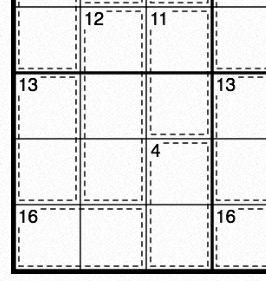The 16-cage in the bottom-left corner has just one cage combination, 7 9, so let's write it in. We don't know which way around the two numbers will be, so we use pencil marks/notes to enter them into both cells - the left-hand cell can contain either 7 or 9, and likewise for the right-hand cell.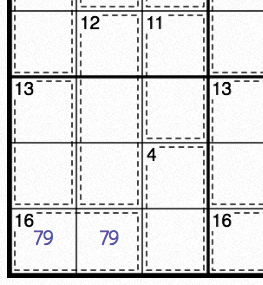Now look at the 13-cage on the left hand side. Its possible combinations are 4 9, 5 8 and 6 7. However, under the rules of Sudoku it can't contain a 7 or a 9, because they already appear elsewhere in the nonet - i.e. in the 16-cage we've just filled in. So, that just leaves 5 8. Let's write that in, again as notes, and also look a bit further up the puzzle: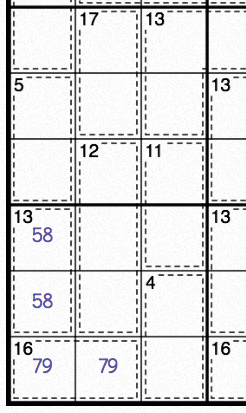The 17-cage at the top of that section also has a single combination, 8 9. Under the rules of Sudoku, that means there can't be another 8 or 9 anywhere else in that column. Looking down at the 16-cage again, that means its right-hand cell can't contain the 9, so it must contain the 7, leaving the left-hand cell containing the 9. Let's write all that in: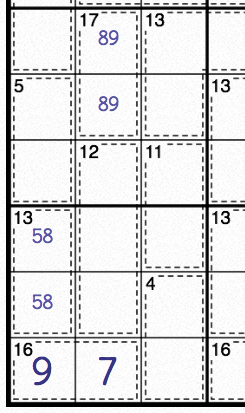As you can see, it's very important to understand cage combinations, and finding cages with just a single combination can be a very effective way to start solving a puzzle.

### The 45 Rule

Another important strategy, which forms the basis for many others, is the 45 Rule. At its heart is the rule, from Sudoku, that each row, column and nonet must contain the numbers 1 to 9 only once.

Given this, if you take a single row and add together each of its cells, the answer will always be 1 + 2 + 3 + 4 + 5 + 6 + 7 + 8 + 9, which is 45. The same is true for any single column or nonet - its cells, when solved, will always sum to 45.

In the same vein, two neighbouring rows, columns or nonets sum to 90, three sum to 135, etc.

Now look at the bottom nonet in the puzzle we started above: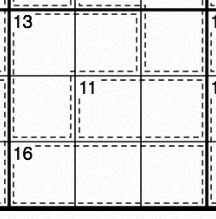We know that the entire nonet's cells must sum to 45. We also know that the small sum in the corner of each cage equals the sum of its cells.

By adding together the cages (13 + 11 + 16), we can work out the sum of the cells shaded in purple below. Given that the purple cells sum to 40, and the purple cells plus the remaining white cell (i.e. all cells in the nonet) sum to 45, the remaining cell must contain 5: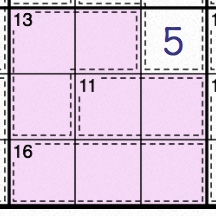This was possible because all-but-one of the nonet's cells are covered by complete cages, leaving a single remaining cell. Finding single remaining cells like this can be another effective way to start solving a Killer Sudoku puzzle.

Daily Killer Sudoku